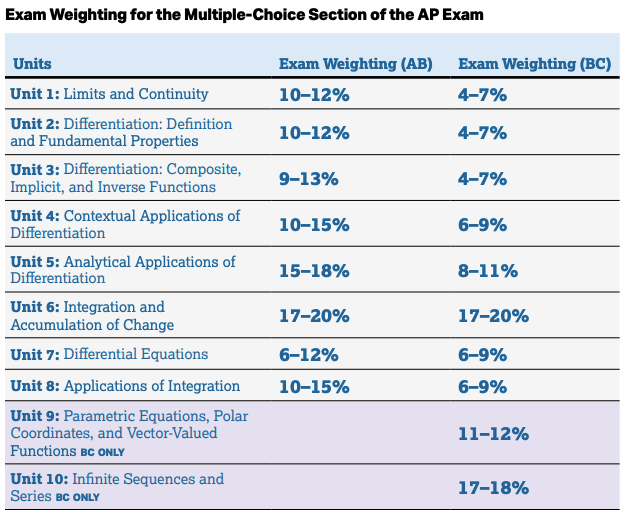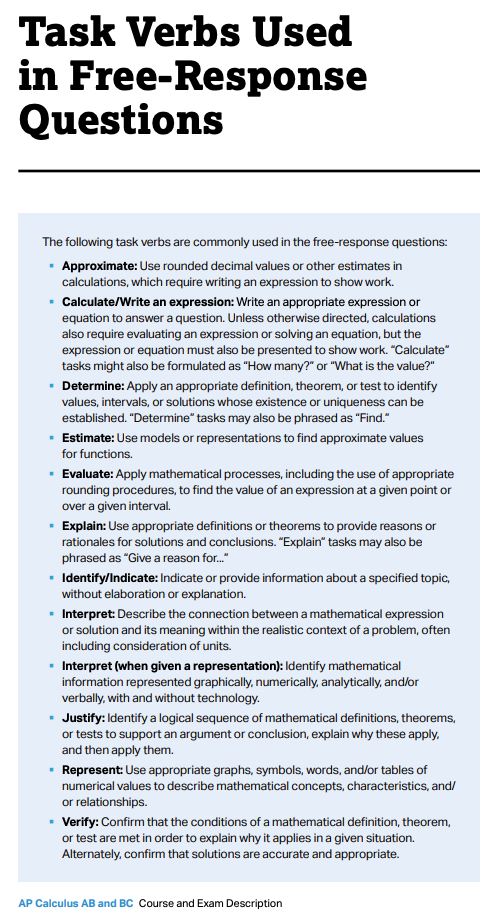Qtr 3 Study Guide - AP Calculus BC

The Quarter 3 Exam will be at 10:15 Thursday March 18, 2021. It will be a 90 minute exam that requires you to download the AP Lockdown browser. You must not be be on any other app. You may not use your Phone. To collaborate, record or communicate any questions or answers to another by any means is dishonorable, and illegal. The best way to study is to practice as if it were a mini AP Exam. Our Qtr 3 Exam is only 90 minutes, but will include all ten units of the AP Calculus Course and Exam Description (CED):The actual AP Calculus Exams will be one of three dates:
1. (Paper, in school) Tuesday May 4, 2021 8:00 AM PDT
2. (Paper, in school) Monday May 24, 2021 8:00 AM PDT
3. (Digital) Wednesday June 9, 2021 9:00 AM PDT

The College Board (that administers the AP exams) has this helpful passage about what should be included in a free response question:

Students are expected to show enough of their work for Readers to follow their line of reasoning. To obtain full credit for the solution to a free-response problem, students must communicate their methods and conclusions clearly. Answers should show enough work so that the reasoning process can be followed throughout the solution. This is particularly important for assessing partial credit. Students may also be asked to use complete sentences to explain their methods or the reasonableness of their answers, or to interpret their results.

For results obtained using the calculator capabilities of plotting, finding zeros, finding the numerical derivative or integral, students are required to write the setup (e.g., the equation being solved, or the derivative or definite integral being evaluated) that leads to the solution, along with the result produced by the calculator.

For example, if the student is asked to find the area of a region, the student is expected to show a definite integral (i.e., the setup) and the answer. The student need not compute the antiderivative; the calculator may be used to calculate the value of the definite integral without further explanation.

For solutions obtained using the calculator capabilities, students must also show the mathematical steps that lead to the answer; a calculator result is not sufficient. For example, if the student is asked to find a relative minimum value of a function, the student is expected to use calculus and show the mathematical steps that lead to the answer. It is not sufficient to graph the function or use a built-in minimum folder.

When a student is asked to justify an answer, the justification must include mathematical reasons, not merely calculator results. Functions, graphs, tables, or other objects that are used in a justification should be clearly identified.

Here is a check list of the topics:

• Logistic and Exponential Modeling
• Partial Fractions
• Arc Length
• Area of a Surface of Revolution
• Basic Integration Rules (page 522)
• Integration by Parts (Ultra-Violet Voodu)
• Trigonometric Integrals (odd and even powers of sine and cosine)
• Integration Using Partial Fractions
• Indeterminate Forms and L’Hôpital’s Rule
• Improper Integrals
• Sequences
• Properties of limits
• Squeeze them for limits
• Absolute value theorem
• Bounded monotonic serum
• Geometric series and convergence
• Properties of infinite series
• Limit and term of the convergent series there're him
• And term test for divergence only
• The integral test NP series test
• Alternating series
• Alternating series remainder zero
• Direct comparison test
• Limit comparison test
• The ratio test
• Taylor polynomials and approximations
• Power series
• Interval convergence
• Representing functions by power series
• Taylor and Maclaurin series
• Plane Curves and Parametric Equations
• The Calculus of Parametric Curves
• Polar Co-ordinates and Polar Graphs
• Area and Arc Length in Polar Coordinates
• Area Between Polar Curves, Finding dy/dx from polar curves
• Vectors in a plane, Vector Valued Functions
• Differentiation and Integration of Vector-Valued Functions
• Velocity and Acceleration of Vector Valued Functions

1. Watch videos at passAPcalc.com
2. DeltaMath Practice
3. Khan Academy's AP Calculus AB Web site Has a lot of great interactive assignments that provide hints, solutions, and links to videos that explain every topic on the AP Exam. You would want to go over the assignments for the topics listed above and consult our class at KhanAcademy
4. Khan Academy's AP Calculus AB
5. myAP.collegeboard.org AP Classroom now has short Daily Videos for Units 1-6
6. Past Exam Questions from the College Board.
7. Past Exam Answers from Mr Calculus.
8. Past Exam Answers from Skylit.com.
9. Exam Information from the College Board
10. AP Exam Info
11. Example Multiple choice and Free response questions are in the AP Course Description (Exam questions begin around page 228)
12. MC questions from 1969-1998
13. 2008 Multiple choice Questions and answers
14. Video Links from the homework page
15. Videos from the Mathorama Podcast
16. Worksheets from the class Google classroom page.
17. Syllabus has a grade calculator.

It would be good to go over old quizzes, the PPC's at myAp.collegeBoard.org, AP Calc BC at Khan Academy (In particular the solved exams section), as well as your old homework; review what you did well, and learn from any mistakes.

. A helpful course review is this BC Calculus Final Review Packet. Another worksheet that might be helpful is a strategy review: AP Calculus:When you see the words....

The exam category is worth 20% of your grade, and will be curved. (There is a grade calculator in the Syllabus)

Remember to a good night's rest, and eat a healthy breakfast!

Good Luck!